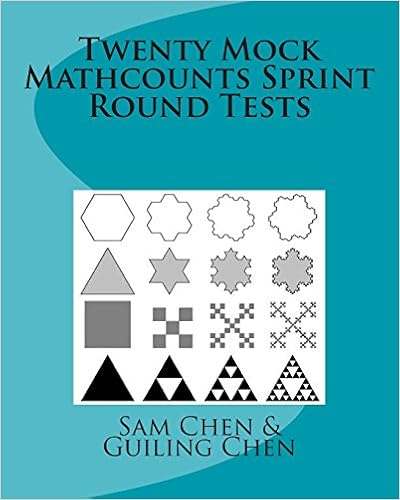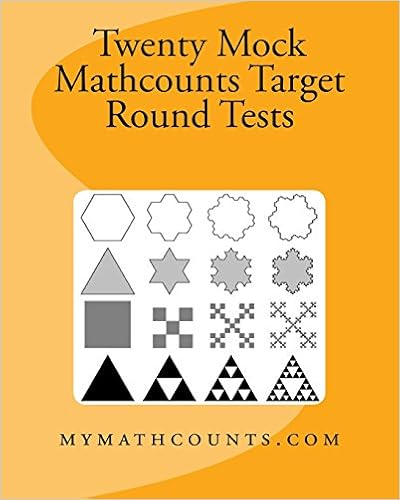HomeTwenty Mock Mathcounts Sprint and Target Practices (Hard Copy Books)

# Twenty Mock Mathcounts Sprint Round and Target Round Practices

Book 1 contains 20 sets of mock Mathcounts Sprint Round Tests.Book 2 contains 20 sets of mock target tests.Each sprint test has 30 problems that you probably won't be able to find in other math books published in the United States. These math problems are great for training school/chapter Mathcounts competitions and State/National Mathcounts competitions and wonderful in training students' math ability and logical reasoning.  Most of the problems in this book are from China middle school math competitions. You can learn a lot from working on these problems, and they are very beneficial. Good luck!

Book 1 contains only answer keys (no solutions). The solutions are posted in our forum (see the link below) for you free to download:
Book 2 contains problems and solutions.

ACKNOWLEDGEMENTS

We like to thank the following Mathcounts coaches who kindly reviewed the manuscripts and made corrections and valuable comments:

Tonya McLawhorn

Mike Whitley

Wendy Hopkins

The Epiprany School

Melanie Simmons

North East Elementary School

Sandra W. Lamm

Greenfield School

Sally Davis

Smyrna Elementary School

1.  Mock Mathcounts Sprint Round Test 1 and answer keys.........1    (Sample pages)

2.   Mock Mathcounts Sprint Round Test 2 and answer keys.........9   (Sample pages)

3.  Mock Mathcounts Sprint Round Test 3 and answer keys.........16

4.  Mock Mathcounts Sprint Round Test 4 and answer keys.........24

5.  Mock Mathcounts Sprint Round Test 5 and answer keys.........32

6.  Mock Mathcounts Sprint Round Test 6 and answer keys.........40

7.   Mock Mathcounts Sprint Round Test 7  and answer keys.........48

8.  Mock Mathcounts Sprint Round Test 8  and answer keys.........55

9.  Mock Mathcounts Sprint Round Test 9  and answer keys.........62

10.  Mock Mathcounts Sprint Round Test 10  and answer keys......70

11.  Mock Mathcounts Sprint Round Test 11 and answer keys......78

12.   Mock Mathcounts Sprint Round Test 12 and answer keys.....86

13.  Mock Mathcounts Sprint Round Test 13 and answer keys......93

14.  Mock Mathcounts Sprint Round Test 14 and answer keys.....100

15.  Mock Mathcounts Sprint Round Test 15 and answer keys.....108

16.  Mock Mathcounts Sprint Round Test 16  and answer keys......117

17.   Mock Mathcounts Sprint Round Test 17 and answer keys......125

18.  Mock Mathcounts Sprint Round Test 18 and answer keys... ...133

19.  Mock Mathcounts Sprint Round Test 19 and answer keys.......141

20.  Mock Mathcounts Sprint Round Test 20 and answer keys.......149 (Sample pages)